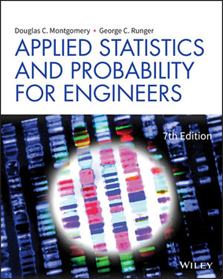# Applied Statistics and Probability for Engineers

Regular price Dhs. 244.00 Sale

7th Edition

By Douglas C. Montgomery, George C. Runger

ISBN: 978-1-119-40036-3
Published: January 2018

DESCRIPTION: provides a practical approach to probability and statistical methods. Students learn how the material will be relevant in their careers by including a rich collection of examples and problem sets that reflect realistic applications and situations. This product focuses on real engineering applications and real engineering solutions while including material on the bootstrap, increased emphasis on the use of p-value, coverage of equivalence testing, and combining p-values. The base content, examples, exercises and answers presented in this product have been meticulously checked for accuracy.

1. The Role of Statistics in Engineering
2. Probability
3. Discrete Random Variables and Probability Distributions
4. Continuous Random Variables and Probability Distributions
5. Joint Probability Distributions
6. Descriptive Statistics
7. Point Estimation of Parameters and Sampling Distributions
8. Statistical Intervals for a Single Sample
9. Tests of Hypotheses for a Single Sample
10. Statistical Inference for Two Samples
11. Simple Linear Regression and Correlation
12. Multiple Linear Regression
13. Design and Analysis of Single-Factor Experiments: The Analysis of Variance
14. Design of Experiments with Several Factors
15. Statistical Quality Control